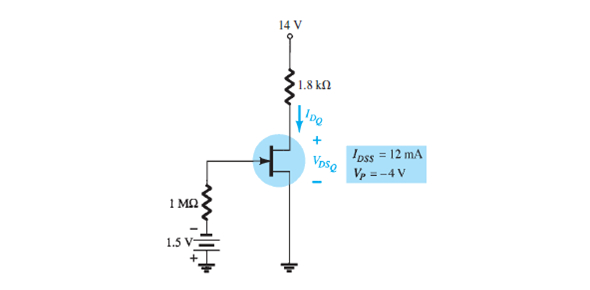# Electronic Devices And Circuit Theory Quiz!

15 Questions | Attempts: 272
ShareSettingsWhat do you know about electronic devices and circuit theory? Do you think you can pass this quiz? For this quiz, you will be required to know how many valence electrons semiconductors have, what is trivalent doped semi-conductor material, the most prevalent semiconductive material in electronic devices, and the purpose of pentavalent impurity. This quiz will shock you; it is so helpful.

• 1.
Semiconductors have how many valence electrons?
• A.

2

• B.

4

• C.

6

• D.

8

• 2.
Trivalent dopants create what type of semiconductor material?
• A.

P-Type

• B.

N-type

• C.

PN Junctions

• 3.
In a trivalent-doped semiconductor material,the majority charge carriers are:
• A.

Holes

• B.

Electrons

• 4.
Valence electrons are:
• A.

In the closest orbit to the nucleus

• B.

In the most distant orbit from the nucleus

• C.

In various orbits around the nucleus

• D.

Not associated with a particular atom

• 5.
The most widely used semiconductive material in electronic devices is:
• A.

Germanium

• B.

Carbon

• C.

Copper

• D.

Silicon

• 6.
The energy band in which free electrons exist is the:
• A.

First band

• B.

Second band

• C.

Conduction band

• D.

Valence band

• 7.
Recombination is when:
• A.

An electron falls into a hole

• B.

A positive and a negative ion bond together

• C.

A valence electron becomes a conduction electron

• D.

A crystal is formed

• 8.
The process of adding an impurity to an intrinsic semiconductor is called:
• A.

Doping

• B.

Recombination

• C.

Atomic modification

• D.

Ionization

• 9.
The purpose of pentavalent impurity is to:
• A.

Reduce the conductivity of silicon

• B.

Increase the number of holes

• C.

Increase the number of free electrons

• D.

Create minority carriers

• 10.
The majority carriers in an n-type semiconductor are:
• A.

Holes

• B.

Valence electrons

• C.

Conduction electrons

• D.

Protons

• 11.
The depletion region consists of:
• A.

Nothing but minority carriers

• B.

Immobile ions

• C.

No majority carriers

• D.

• 12.
The term bias means?
• A.

The ratio of majority carriers to minority carriers

• B.

The amount of current across the doide

• C.

A dc voltage is applied to control the operation of a device

• D.

Neither (A),(B),nor (C)

• 13.
To forward-bias a diode:
• A.

An external voltage is applied that is positive at the anode ana negative at the cathode

• B.

An external voltage is applied that is negative at the anode and positive at the cathode

• C.

An external voltage is applied that is positive at the p region and negative at the n region

• D.

• 14.
When a diode is forward-biased:
• A.

No current is flowing

• B.

Acts like a Open switch

• C.

Current is flowing due to majority carriers only

• D.

The current is produced by both holes and electrons

• 15.
Although current is blocked in reverse bias:
• A.

Resemble like a switch is ON state

• B.

There is a very small current due to minority carriers

• C.

Resistance is lower

## Related TopicsBack to top
×

Wait!
Here's an interesting quiz for you.